Number and Place Value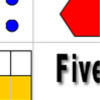Number Match

Age 5 to 11 Challenge Level:

A task which depends on members of the group noticing the needs of others and responding.Representing Numberslive

Age 7 to 11 Challenge Level:

Find as many different ways of representing this number of dots as you can.Planning a School Trip

Age 7 to 11 Challenge Level:

You are organising a school trip and you need to write a letter to parents to let them know about the day. Use the cards to gather all the information you need.Satisfying Four Statements

Age 7 to 11 Challenge Level:

Can you find any two-digit numbers that satisfy all of these statements?Which Scripts?

Age 7 to 11 Challenge Level:

There are six numbers written in five different scripts. Can you sort out which is which?Sitting Round the Party Tables

Age 5 to 11 Challenge Level:

Sweets are given out to party-goers in a particular way. Investigate the total number of sweets received by people sitting in different positions.The Deca Tree

Age 7 to 11 Challenge Level:

Find out what a Deca Tree is and then work out how many leaves there will be after the woodcutter has cut off a trunk, a branch, a twig and a leaf.Take Three Numbers

Age 7 to 11 Challenge Level:

What happens when you add three numbers together? Will your answer be odd or even? How do you know?A Mixed-up Clock

Age 7 to 11 Challenge Level:

There is a clock-face where the numbers have become all mixed up. Can you find out where all the numbers have got to from these ten statements?Number Differences

Age 7 to 11 Challenge Level:

Place the numbers from 1 to 9 in the squares below so that the difference between joined squares is odd. How many different ways can you do this?Swimming Pool

Age 5 to 11 Challenge Level:

In this problem, we're investigating the number of steps we would climb up or down to get out of or into the swimming pool. How could you number the steps below the water?How Would We Count?

Age 5 to 11 Challenge Level:

An activity centred around observations of dots and how we visualise number arrangement patterns.First Connect Three

Age 7 to 14 Challenge Level:

The idea of this game is to add or subtract the two numbers on the dice and cover the result on the grid, trying to get a line of three. Are there some numbers that are good to aim for?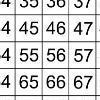That Number Square!

Age 5 to 11 Challenge Level:

Exploring the structure of a number square: how quickly can you put the number tiles in the right place on the grid?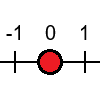Tug Harder!

Age 7 to 11 Challenge Level:

In this game, you can add, subtract, multiply or divide the numbers on the dice. Which will you do so that you get to the end of the number line first?Round the Four Dice

Age 7 to 11 Challenge Level:

This activity involves rounding four-digit numbers to the nearest thousand.Sea Level

Age 7 to 11 Challenge Level:

The picture shows a lighthouse and many underwater creatures. If you know the markings on the lighthouse are 1m apart, can you work out the distances between some of the different creatures?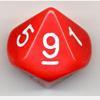Reasoned Roundinglive

Age 7 to 14 Challenge Level:

Four strategy dice games to consolidate pupils' understanding of rounding.Magic Vs

Age 7 to 11 Challenge Level:

Can you put the numbers 1-5 in the V shape so that both 'arms' have the same total?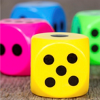Dicey Operations in Line

Age 7 to 11 Challenge Level:

Who said that adding, subtracting, multiplying and dividing couldn't be fun?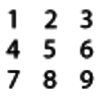Four-digit Targets

Age 7 to 11 Challenge Level:

You have two sets of the digits 0 – 9. Can you arrange these in the five boxes to make four-digit numbers as close to the target numbers as possible?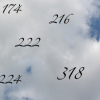Count Me Inlive

Age 7 to 11 Challenge Level:

How do you know whether you will reach these numbers when you count in steps of six from zero?The Remainders Game

Age 7 to 14 Challenge Level:

Play this game and see if you can figure out the computer's chosen number.Coded Hundred Square

Age 7 to 11 Challenge Level:

This 100 square jigsaw is written in code. It starts with 1 and ends with 100. Can you build it up?Nice or Nasty

Age 7 to 14 Challenge Level:

There are nasty versions of this dice game but we'll start with the nice ones...Space Distanceslive

Age 7 to 11 Challenge Level:

This task focuses on distances travelled by the asteroid Florence. It's an opportunity to work with very large numbers.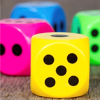Dicey Operations

Age 11 to 14 Challenge Level:

Who said that adding, subtracting, multiplying and dividing couldn't be fun?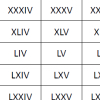Roman Numeralslive

Age 7 to 11 Challenge Level:

Can you find some examples when the number of Roman numerals is fewer than the number of Arabic numerals for the same number?Number Lines in Disguise

Age 7 to 14 Challenge Level:

Some of the numbers have fallen off Becky's number line. Can you figure out what they were?Three Neighbours

Age 7 to 11 Challenge Level:

Look at three 'next door neighbours' amongst the counting numbers. Add them together. What do you notice?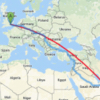What Distance?live

Age 7 to 11 Challenge Level:

This task gives an opportunity to perform some subtractions in a slightly realistic situation.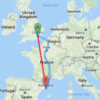Ordering Journeyslive

Age 7 to 11 Challenge Level:

How would you put these journey lengths in order? Give a bit of a place value challenge with subtractions set out not as a calculation.Square Subtraction

Age 7 to 11 Challenge Level:

Look at what happens when you take a number, square it and subtract your answer. What kind of number do you get? Can you prove it?